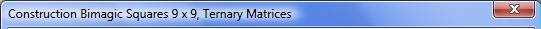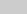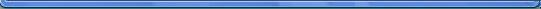Office Applications and Entertaiment, Magic SquaresForm9 About the Author

Bimagic Squares 9 x 9, Interactive Solution based on Ternary Matrices

Introduction

A Magic Square is bimagic if it remains magic after each of the numbers have been squared. It has been proven that the smallest order of Bimagic Squares is 8.

The Magic Sum of the squared numbers is n(n2 + 1)(2n2 + 1)/6 = s1(2n2 +1)/3 with s1 the magic sum of the original square.

John Hendricks devellopped a Class of 9th order bimagic squares - based on the ‘digital equation’ method - which can be decomposed into 4 regular grids based on the ternar representation of numbers (0 = 0000, 1 = 0001, 2 = 0002, 3 = 0010 ...
80 = 2222).

Any Magic Square of the 9th order with the numbers 1 ... 81 can be written as G1 + 3 * G2 + 9 * G3 + 27 * G4 + , where the matrices G1, G2, G3 and G4 - further referred to as grids - contain only the numbers 0, 1 and 2.

The Base Grids G1, G2, G3 and G4 - suitable for the construction of bimagic squares - can be viewed in the form below by selecting G1, G2, G3 or G4 with the left selection button and pressing the button 'Shw G'.

The grids can be put in random order and the numbers 0, 1 and 2 in each grid can be swapped.

The construction method based on the principles described above has been applied in following Interactive Solution:G1   G2    G3   G4

 1 2 3 4 5 6 1 2 3 4 5 6 1 2 3 4 5 6 1 2 3 4 5 6 G1 G2 G3 G4

Sequence:

 1 2 3 4 1 2 3 4 1 2 3 4 1 2 3 4Procedure:

1. Select the Ternary Matrices with the four upper left selection buttons and validate the selection with the button 'Validate'.

2. The selected Matrices can be viewed by selecting G1, G2, G3 or G4 with the left selection button and pressing the button 'Shw G'.

3. Define the sequence with the applicable selection buttons (select 1, 2 ... 4 for G1, G2 ... G4).

4. Press the button ‘Shw Sqr 1’ to calculate and visualise the resulting Bimagic Square.

5. Press the button ‘Shw Sqr 2’ to calculate and visualise the corresponding squared numbers.

The 4! = 24 permutations of 64 = 1296 possible grids results in 24 * 1296 = 31104 possible Bimagic Squares.

Have Fun!About the Author